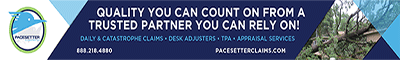#### Building Materials - Circuit Breaker

##### Replacement Cost
\$
.00
Building Materials - Circuit Breaker Depreciation Rate: 3.33% per year
Keywords: gfci, afci, circuit breaker, ground-fault, circuit interrupter, ground fault
##### DEPRECIATION FORMULA
ACV = RCV - (DPR * RCV * AGE)

##### EQUATION VARIABLES
ACV = Actual Cash Value (Depreciated Value)
AGE = Age of Item (Years)
RCV = Replacement Cash Value (Cost to Purchase Now)
DPR = Depreciation Rate (% per Year)